{literal} {/literal}
{php} if(\$GLOBALS['lang'] == 'zh'){ \$sql = "SELECT * FROM ".\$GLOBALS['eces']->table('firm_products')." WHERE FirmID = '". \$GLOBALS['FrimID']."' "; }elseif(\$GLOBALS['lang'] == 'tw'){ \$sql = "SELECT * FROM ".\$GLOBALS['eces']->table('firm_products_tw')." WHERE FirmID = '". \$GLOBALS['FrimID']."' "; }else{ \$sql = "SELECT * FROM ".\$GLOBALS['eces']->table('firm_products_en')." WHERE FirmID = '". \$GLOBALS['FrimID']."' "; } \$sql .= "ORDER BY `addTime` DESC LIMIT 0,12 "; \$RS = \$GLOBALS['dbes']->getAll(\$sql); foreach(\$RS AS \$v){ if(!empty(\$v[PicPath])){ \$picimg = \$v[PicPath] ; }else{ \$picimg = \$GLOBALS['tpl']['img']."imgjs/erreso.gif"; } if(\$idd < 6){ \$contenet .= ''.\$v[Name].'
'; }else{ \$contenet2 .= ''.\$v[Name].'
'; } \$idd++; } echo '
• '.\$contenet.'
• '.\$contenet2.'
• '; {/php}
{literal} {/literal}
{php} if(\$GLOBALS['lang'] == 'zh'){ \$sql = "SELECT cat_id,cat_name,parent_id FROM ".\$GLOBALS['eces']->table('category_zh')." WHERE FirmID = '". \$GLOBALS['FrimID']."' AND parent_id = '0' ORDER BY `sort_order` ASC "; }elseif(\$GLOBALS['lang'] == 'tw'){ \$sql = "SELECT cat_id,cat_name,parent_id FROM ".\$GLOBALS['eces']->table('category_tw')." WHERE FirmID = '". \$GLOBALS['FrimID']."' AND parent_id = '0' ORDER BY `sort_order` ASC "; }else{ \$sql = "SELECT cat_id,cat_name,parent_id FROM ".\$GLOBALS['eces']->table('category_en')." WHERE FirmID = '". \$GLOBALS['FrimID']."' AND parent_id = '0' ORDER BY `sort_order` ASC "; } \$RS = \$GLOBALS['dbes']->getAll(\$sql); \$count = 1; foreach(\$RS as \$key => \$v){ \$contenall .= '
• '.\$v[cat_name].'
• '; } echo \$contenall; {/php}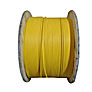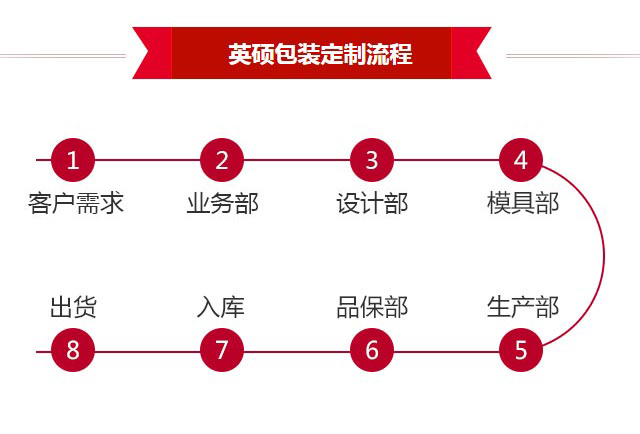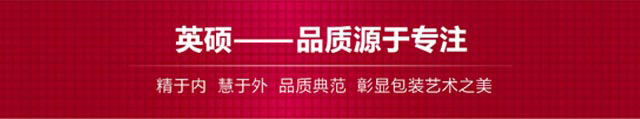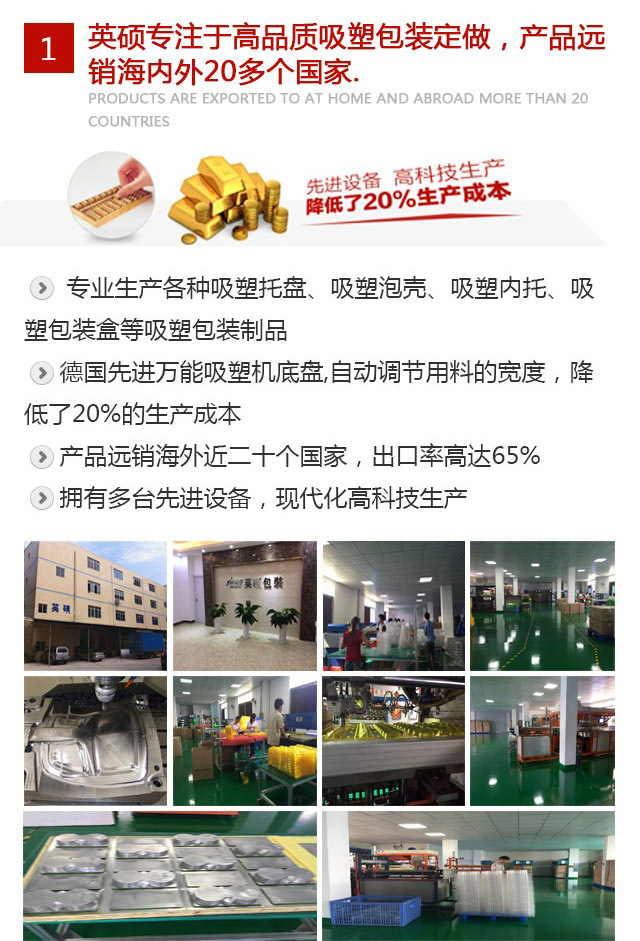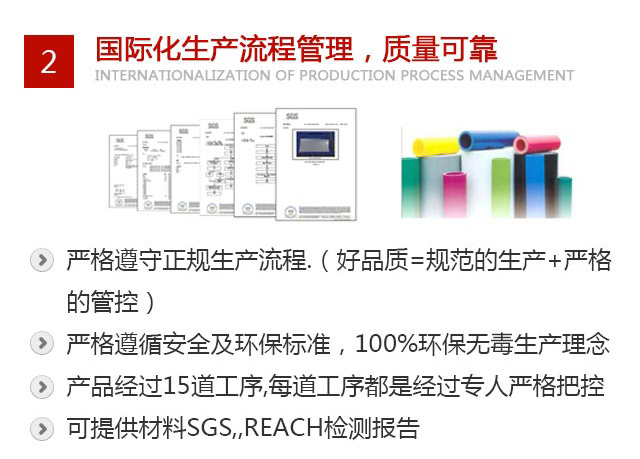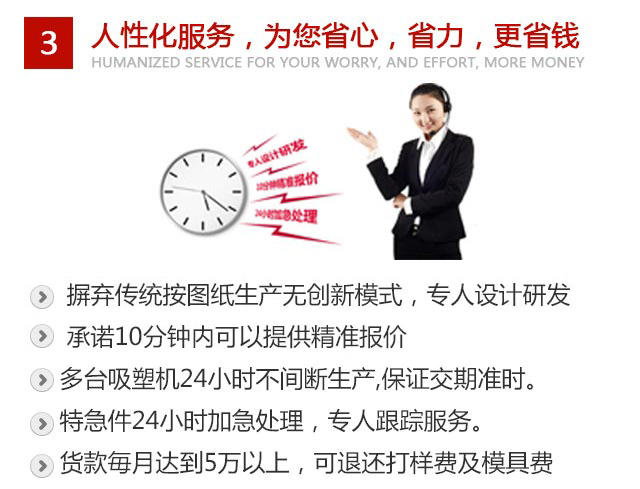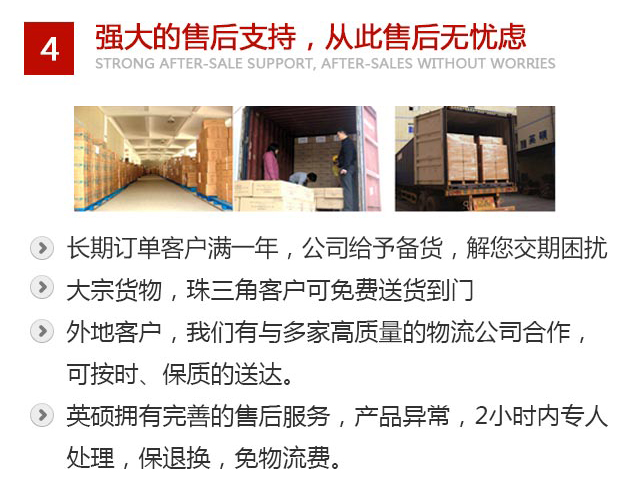• 公司新闻
• 行业新闻
{literal} {/literal}